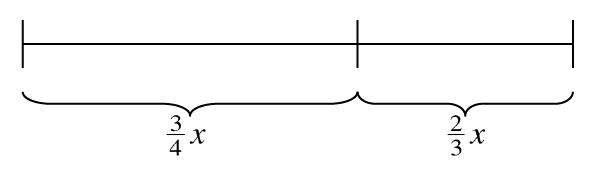### Home > CC3MN > Chapter 3 > Lesson 3.1.3 > Problem3-32

3-32.

Combine like terms in each part below.

1. Liha has three $x^2$ -tiles, two $x$-tiles, and eight unit tiles, while Makulata has five $x^2$-tiles and two unit tiles. At the end of class, they put their pieces together to give to Ms. Singh. Write an algebraic expression for each student's tiles and find the sum of their pieces.

Write down Liha's and Makulata's tile pieces as expressions.

Liha $3x^2+2x+8$
Makulata $5x^2+2$

Now add the two together.

$3x^2+2x+8+5x^2+2=8x^2+2x+10$

2. Simplify the expression $4x+6x^2-11x+2+x^2-19$.

Add like terms and then simplify.

3. Write the length of the line below as a sum. Then combine like terms.Write the two terms down as an expression.

$\frac{3}{4}{\it x} + \frac{2}{3}{\it x}$

Convert both fractions to a common denominator before combining like terms.